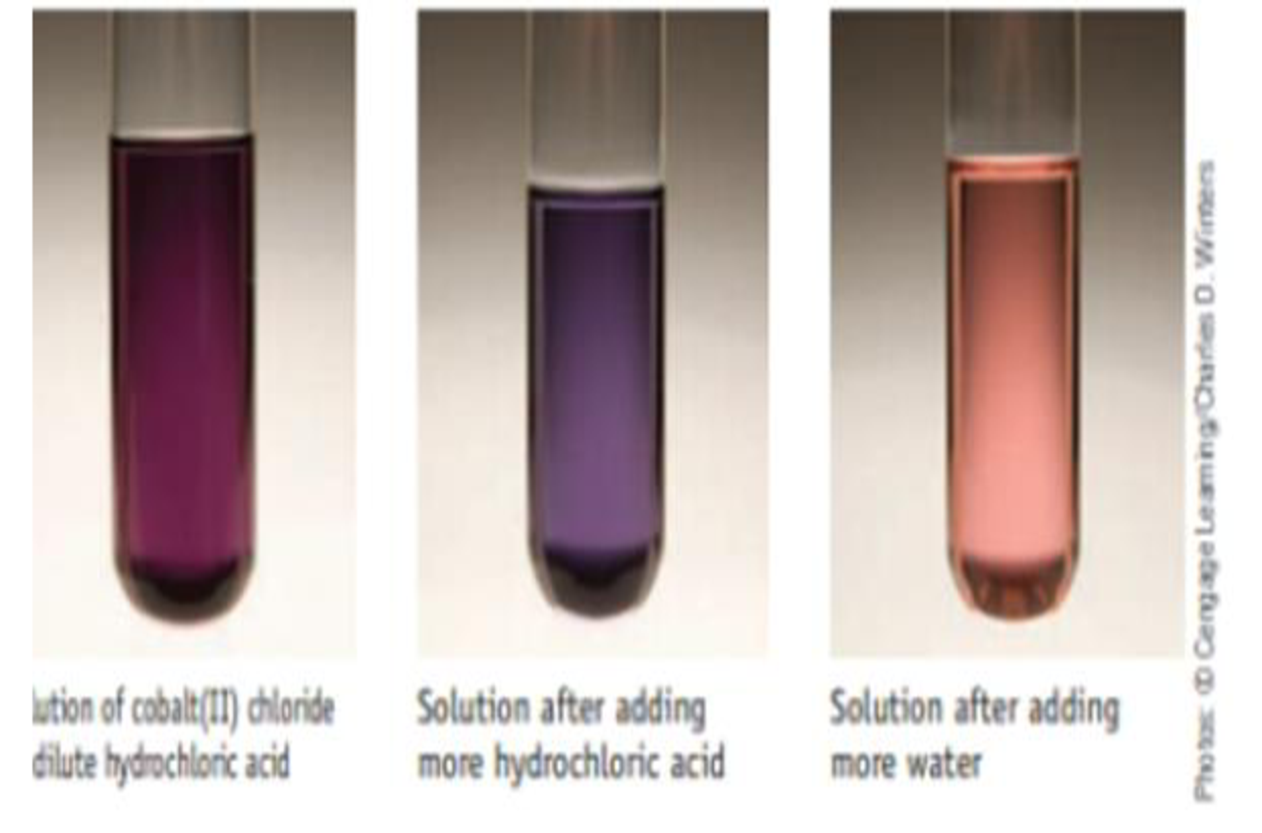Chapter 15, Problem 74SCQ

Chapter
Section
Textbook Problem

The chapter opening photograph (page 670) showed how the cobalt(II) chloride equilibrium responded to temperature changes. (a) Look back at that photograph. Is the conversion of the red cation to the blue anion exothermic or endothermic? (b) If hydrochloric acid is added to the violet mixture of cobalt(II) ions shown below, the blue CoCl42− ion is favored. If water is then added to the mixture, a red solution favoring [Co(H2O)]2+ results. Explain these observations in terms of Le Chatelier’s principle.(c) How do these observations prove the reaction is reversible?

(a)

Interpretation Introduction

Interpretation:

The conversion of red cation to the blue anion with temperature changes for the given reaction is exothermic or endothermic has to be identified.

Concept Introduction:

Le Chatelier’s principle: If equilibrium is disturbed by changing conditions, the system will moves the equilibrium to reverse the change.

Factor’s that effect chemical equilibria:

Concentration – Equilibrium will be affected by changing the concentration of reactant or product. If we increase the concentration of reactant system will try to reverse the change by favouring forward reaction and thus increase the concentration of products. Likewise adding products increase yield of reactants.

Temperature – When the temperature increases equilibrium will shift in the endothermic direction, in the direction that absorbs heat. When the temperature decreases equilibrium will shift in the exothermic direction, in the direction that releases heat.

Pressure – If the reaction consists of only liquid and solid reactants and products, pressure has no effect in the equilibrium.

In gas reactions if the number of moles has no change then there will be no effect by pressure on equilibrium.

If pressure increases then equilibrium will shift to the direction having less number of molecules and if pressure decreases system will shift to the direction having more number of molecules.

Explanation

Given reaction:

[Co(H2.O)6]+2(aq)+4Cl-(aq)[COCl4]2-(aq)+6H2O(l)red&#

(b)

Interpretation Introduction

Interpretation:

The observations when HCl is added to the violet solution has to be given.

Concept Introduction:

Le Chatelier’s principle: If equilibrium is disturbed by changing conditions, the system will moves the equilibrium to reverse the change.

Factor’s that effect chemical equilibria:

Concentration – Equilibrium will be affected by changing the concentration of reactant or product. If we increase the concentration of reactant system will try to reverse the change by favouring forward reaction and thus increase the concentration of products. Likewise adding products increase yield of reactants.

Temperature – When the temperature increases equilibrium will shift in the endothermic direction, in the direction that absorbs heat. When the temperature decreases equilibrium will shift in the exothermic direction, in the direction that releases heat.

Pressure – If the reaction consists of only liquid and solid reactants and products, pressure has no effect in the equilibrium.

In gas reactions if the number of moles has no change then there will be no effect by pressure on equilibrium.

If pressure increases then equilibrium will shift to the direction having less number of molecules and if pressure decreases system will shift to the direction having more number of molecules.

(c)

Interpretation Introduction

Interpretation:

The observations mentioned in the given reaction are reversible or not has to be proved.

Concept Introduction:

Le Chatelier’s principle: If equilibrium is disturbed by changing conditions, the system will moves the equilibrium to reverse the change.

Factor’s that effect chemical equilibria:

Concentration – Equilibrium will be affected by changing the concentration of reactant or product. If we increase the concentration of reactant system will try to reverse the change by favouring forward reaction and thus increase the concentration of products. Likewise adding products increase yield of reactants.

Temperature – When the temperature increases equilibrium will shift in the endothermic direction, in the direction that absorbs heat. When the temperature decreases equilibrium will shift in the exothermic direction, in the direction that releases heat.

Pressure – If the reaction consists of only liquid and solid reactants and products, pressure has no effect in the equilibrium.

In gas reactions if the number of moles has no change then there will be no effect by pressure on equilibrium.

If pressure increases then equilibrium will shift to the direction having less number of molecules and if pressure decreases system will shift to the direction having more number of molecules.

Still sussing out bartleby?

Check out a sample textbook solution.

See a sample solution

The Solution to Your Study Problems

Bartleby provides explanations to thousands of textbook problems written by our experts, many with advanced degrees!

Get Started

Find more solutions based on key concepts